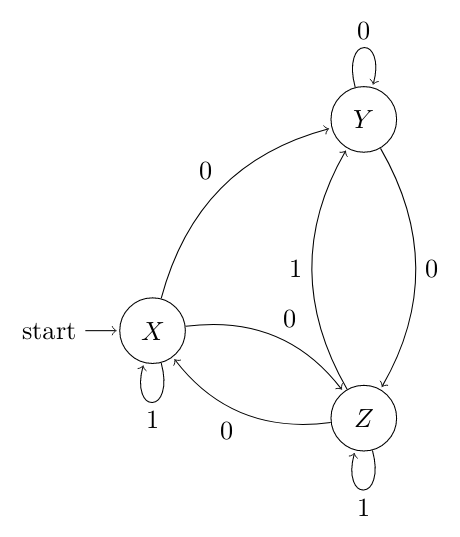# GATE | Gate IT 2005 | Question 37

• Difficulty Level : Hard
• Last Updated : 06 Jun, 2019

Consider the non-deterministic finite automaton (NFA) shown in the figure.State X is the starting state of the automaton. Let the language accepted by the NFA with Y as the only accepting state be L1. Similarly, let the language accepted by the NFA with Z as the only accepting state be L2. Which of the following statements about L1 and L2 is TRUE?

Correction in Question:
There is an edge from Z->Y labeled 0 and another edge from Y->Z labeled 1 – in place of double arrowed and no arrowed edges.

Attention reader! Don’t stop learning now.  Practice GATE exam well before the actual exam with the subject-wise and overall quizzes available in GATE Test Series Course.

Learn all GATE CS concepts with Free Live Classes on our youtube channel.

(A) L1 = L2
(B) L1 ⊂ L2
(C) L2 ⊂ L1
(D) None of the above

Explanation: According to Arden’s Theorem,

For y as final state:

`Y = X0 + Y0 + Z1 `

For z as final state:

`Z = X0 + Y0 + Z1 `

Hence,

`L(Y) = L(Z) `

So, option (A) is correct.

My Personal Notes arrow_drop_up